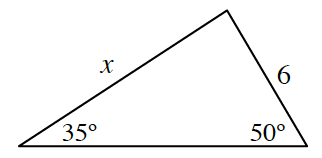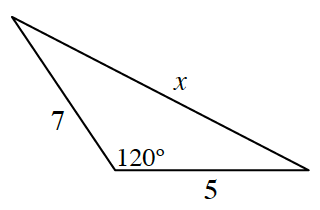### Home > PC > Chapter 2 > Lesson 2.3.4 > Problem2-117

2-117.

Find the value of $x$ for each triangle.

1.Find the 3rd angle. Should you use the Law of Sines or Cosines to solve for $x$?

Law of Sines would be easiest.Should you use the Law of Sines or the Law of Cosines to find $x$? Why?

Use the Law of Cosines, because the Law of Sines needs an angle and a value of an opposite side.